# Loss Functions¶

Generally, a loss function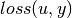is to measure the loss between the predicted output u and the desired response y. In this package, all loss functions are instances of the abstract type Loss, defined as below:

# N is the number of dimensions of each predicted output
# 0 - scalar
# 1 - vector
# 2 - matrix, ...
#
abstract Loss{N}

typealias UnivariateLoss Loss{0}
typealias MultivariateLoss Loss{1}


## Common Methods¶

### Methods for Univariate Loss¶

Each univariate loss function implements the following methods:

value(loss, u, y)

Compute the loss value, given the predicted output u and the desired response y.

deriv(loss, u, y)

Compute the derivative w.r.t. u.

value_and_deriv(loss, u, y)

Compute both the loss value and derivative (w.r.t. u) at the same time.

Note

This can be more efficient than calling value and deriv respectively, when you need both the value and derivative.

### Methods for Multivariate Loss¶

Each multivariate loss function implements the following methods:

value(loss, u, y)

Compute the loss value, given the predicted output u and the desired response y.

grad!(loss, g, u, y)

Compute the gradient w.r.t. u, and write the results to g. This function returns g.

Note

g is allowed to be the same as u, in which case, the content of u will be overrided by the derivative values.

value_and_grad!(loss, g, u, y)

Compute both the loss value and the derivative w.r.t. u at the same time. This function returns (v, g), where v is the loss value.

Note

g is allowed to be the same as u, in which case, the content of u will be overrided by the derivative values.

For multivariate loss functions, the package also provides the following two generic functions for convenience.

grad(loss, u, y)

Compute and return the gradient w.r.t. u.

value_and_grad(loss, u, y)

Compute and return both the loss value and the gradient w.r.t. u, and return them as a 2-tuple.

Both grad and value_and_grad are thin wrappers of the type-specific methods grad! and value_and_grad!.

## Predefined Loss Functions¶

This package provides a collection of loss functions that are commonly used in machine learning practice.

### Absolute Loss¶

The absolute loss, defined below, is often used for real-valued robust regression: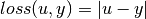immutable AbsLoss <: UnivariateLoss end


### Squared Loss¶

The squared loss, defined below, is widely used in real-valued regression: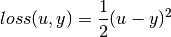immutable SqrLoss <: UnivariateLoss end


### Quantile Loss¶

The quantile loss, defined below, is used in models for predicting typical values. It can be considered as a skewed version of the absolute loss.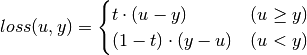immutable QuantileLoss <: UnivariateLoss
t::Float64

function QuantileLoss(t::Real)
...
end
end


### Huber Loss¶

The Huber loss, defined below, is used mostly in real-valued regression, which is a smoothed version of the absolute loss.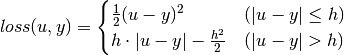immutable HuberLoss <: UnivariateLoss
h::Float64

function HuberLoss(h::Real)
...
end
end


### Hinge Loss¶

The hinge loss, defined below, is mainly used for large-margin classification (e.g. SVM).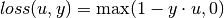immutable HingeLoss <: UnivariateLoss end


### Smoothed Hinge Loss¶

The smoothed hinge loss, defined below, is a smoothed version of the hinge loss, which is differentiable everywhere.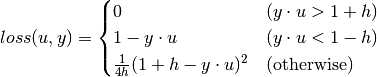immutable SmoothedHingeLoss <: UnivariateLoss
h::Float64

function SmoothedHingeLoss(h::Real)
...
end
end


### Logistic Loss¶

The logistic loss, defined below, is the loss used in the logistic regression.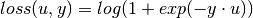immutable LogisticLoss <: UnivariateLoss end


### Sum Loss¶

The package provides the SumLoss type that turns a univariate loss into a multivariate loss. The definition is given below: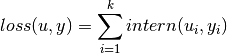Here, intern is the internal univariate loss.

immutable SumLoss{L<:UnivariateLoss} <: MultivariateLoss
intern::L
end

SumLoss{L<:UnivariateLoss}(loss::L) = SumLoss{L}(loss)


Moreover, recognizing that sum of squared difference is very widely used. We provide a SumSqrLoss as a typealias as follows:

typealias SumSqrLoss SumLoss{SqrLoss}
SumSqrLoss() = SumLoss{SqrLoss}(SqrLoss())


### Multinomial Logistic Loss¶

The multinomial logistic loss, defined below, is the loss used in multinomial logistic regression (for multi-way classification).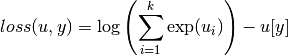Here, y is the index of the correct class.

immutable MultiLogisticLoss <: MultivariateLoss